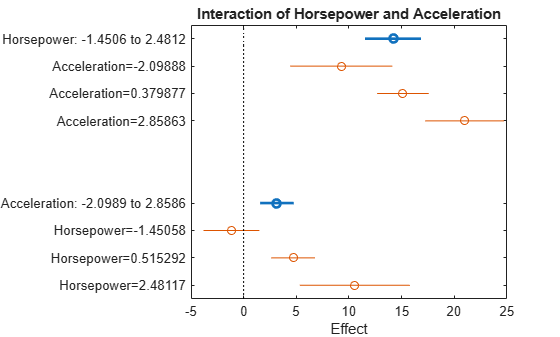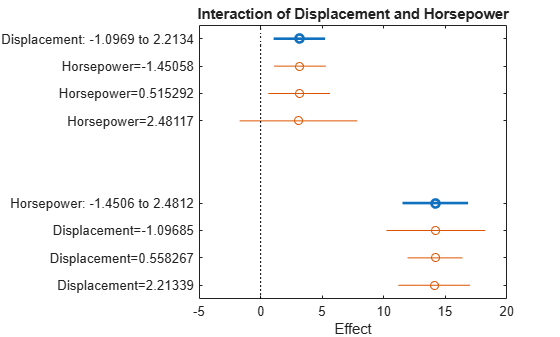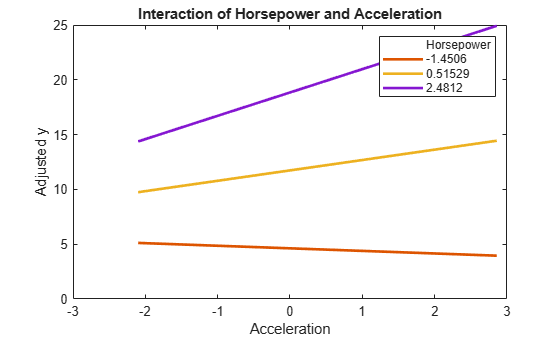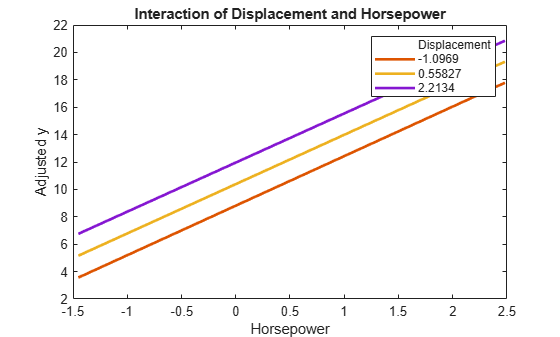Documentation

# plotInteraction

Plot interaction effects of two predictors in linear regression model

## Syntax

``plotInteraction(mdl,var1,var2)``
``plotInteraction(mdl,var1,var2,ptype)``
``h = plotInteraction(___)``

## Description

example

````plotInteraction(mdl,var1,var2)` creates a plot of the main effects of the two selected predictors `var1` and `var2` and their conditional effects in the linear regression model `mdl`. Horizontal lines through the effect values indicate their 95% confidence intervals.```

example

````plotInteraction(mdl,var1,var2,ptype)` specifies the plot type `ptype`. For example, if `ptype` is `'predictions'`, then `plotInteraction` plots the adjusted response function as a function of the second predictor, with the first predictor fixed at specific values. For details, see Conditional Effect.```
````h = plotInteraction(___)` returns line objects using any of the input argument combinations in the previous syntaxes. Use `h` to modify the properties of a specific line after you create the plot. For a list of properties, see Line Properties.```

## Examples

collapse all

Fit a model with an interaction term and create an interaction plot that shows the main effects and conditional effects.

Using the data in the `carsmall` data set, create response values that include an interaction term. First, load the data set and normalize the predictor data.

```load carsmall Acceleration = normalize(Acceleration); Horsepower = normalize(Horsepower); Displacement = normalize(Displacement);```

Define a response variable that includes the interaction term `Acceleration*Horsepower`.

`y = Acceleration + 4*Horsepower + Acceleration.*Horsepower + Displacement;`

Add some noise to the response values.

```rng('default') % For reproducibility y = y + normrnd(10,0.25*nanstd(y),size(y));```

Create a table that includes the predictor data and response values.

`tbl = table(Acceleration,Horsepower,Displacement,y);`

Fit a linear regression model.

`mdl = fitlm(tbl,'y ~ Acceleration + Horsepower + Acceleration*Horsepower + Displacement + Horsepower*Displacement')`
```mdl = Linear regression model: y ~ 1 + Acceleration*Horsepower + Horsepower*Displacement Estimated Coefficients: Estimate SE tStat pValue __________ _______ _________ __________ (Intercept) 9.8652 0.16177 60.982 8.587e-77 Acceleration 0.63726 0.1626 3.9191 0.00016967 Horsepower 3.6168 0.34 10.638 9.273e-18 Displacement 0.95032 0.31828 2.9858 0.0036144 Acceleration:Horsepower 0.60108 0.1851 3.2473 0.0016209 Horsepower:Displacement -0.0096069 0.20947 -0.045863 0.96352 Number of observations: 99, Error degrees of freedom: 93 Root Mean Squared Error: 1.07 R-squared: 0.93, Adjusted R-Squared: 0.927 F-statistic vs. constant model: 249, p-value = 3.3e-52 ```

`pValue` of the interaction term `Acceleration*Horsepower` is very small, meaning that the interaction term is statistically significant.

Create an interaction plot that shows the main effects and conditional effects of `Horsepower` and `Acceleration`.

`plotInteraction(mdl,'Horsepower','Acceleration')`For each predictor, the main effect point and its conditional effect points are not vertically aligned. Therefore, you cannot find any vertical lines that pass through the confidence intervals of the main and conditional effect points for each predictor. This plot indicates the existence of interaction effects on the response variable.

For comparison, create an interaction plot for `Displacement` and `Horsepower`. This p-value of this interaction term (`Displacement*Horsepower`) is large, meaning that the interaction term is not statistically significant.

`plotInteraction(mdl,'Displacement','Horsepower')`For each predictor, the main effect point and its conditional effect points are aligned vertically. This plot indicates no interaction.

Fit a model with an interaction term and create an interaction plot of adjusted response curves.

Using the data in the `carsmall` data set, create response values that include an interaction term. First, load the data set and normalize the predictor data.

```load carsmall Acceleration = normalize(Acceleration); Horsepower = normalize(Horsepower); Displacement = normalize(Displacement);```

Define a response variable that includes the interaction term `Acceleration*Horsepower`.

`y = Acceleration + 4*Horsepower + Acceleration.*Horsepower + Displacement;`

Add some noise to the response values.

```rng('default') % For reproducibility y = y + normrnd(10,0.25*nanstd(y),size(y));```

Create a table that includes the predictor data and response values.

`tbl = table(Acceleration,Horsepower,Displacement,y);`

Fit a linear regression model.

`mdl = fitlm(tbl,'y ~ Acceleration + Horsepower + Acceleration*Horsepower + Displacement + Horsepower*Displacement')`
```mdl = Linear regression model: y ~ 1 + Acceleration*Horsepower + Horsepower*Displacement Estimated Coefficients: Estimate SE tStat pValue __________ _______ _________ __________ (Intercept) 9.8652 0.16177 60.982 8.587e-77 Acceleration 0.63726 0.1626 3.9191 0.00016967 Horsepower 3.6168 0.34 10.638 9.273e-18 Displacement 0.95032 0.31828 2.9858 0.0036144 Acceleration:Horsepower 0.60108 0.1851 3.2473 0.0016209 Horsepower:Displacement -0.0096069 0.20947 -0.045863 0.96352 Number of observations: 99, Error degrees of freedom: 93 Root Mean Squared Error: 1.07 R-squared: 0.93, Adjusted R-Squared: 0.927 F-statistic vs. constant model: 249, p-value = 3.3e-52 ```

`pValue` of the interaction term `Acceleration*Horsepower` is very small, meaning that the interaction term is statistically significant.

Create an interaction plot that shows the adjusted response function as a function of `Acceleration`, with `Horsepower` fixed at specific values.

`plotInteraction(mdl,'Horsepower','Acceleration','predictions')`The curves are not parallel. This plot indicates interactions between the predictors.

For comparison, create an interaction plot for the `Displacement` and `Horsepower`. The p-value of this interaction term (`Displacement*Horsepower`) is large, meaning that the interaction term is not statistically significant.

`plotInteraction(mdl,'Displacement','Horsepower','predictions')`The curves are parallel, indicating no interaction.

## Input Arguments

collapse all

Linear regression model object, specified as a `LinearModel` object created by using `fitlm` or `stepwiselm`, or a `CompactLinearModel` object created by using `compact`.

First variable for the plot, specified as a character vector or string array of the variable name in `mdl.VariableNames` (`VariableNames` property of `mdl`), or a positive integer representing the index of a variable in `mdl.VariableNames`.

Data Types: `char` | `string` | `single` | `double`

Second variable for the plot, specified as a character vector or string array of the variable name in `mdl.VariableNames` (`VariableNames` property of `mdl`), or a positive integer representing the index of a variable in `mdl.VariableNames`.

Data Types: `char` | `string` | `single` | `double`

Plot type, specified as one of these values:

• `'effects'``plotInteraction` creates a plot of the main effects of the two selected predictors `var1` and `var2` and their conditional effects. Horizontal lines through the effect values indicate their 95% confidence intervals.

• `'predictions'``plotInteraction` plots the adjusted response function as a function of `var2`, with `var1` fixed at specific values.

For details, see Main Effect and Conditional Effect.

## Output Arguments

collapse all

Line objects, returned as a vector. Use dot notation to query and set properties of the line objects. For details, see Line Properties.

If the plot type is `'effects'` (default), `h(1)` corresponds to the circles that represent the main effect estimates, and `h(2)` and `h(3)` correspond to the 95% confidence intervals for the two main effects. The remaining entries in `h` correspond to the conditional effects and their confidence intervals. The line objects associated with the main effects have the tag `'main'`. The line objects associated with the conditional effects of `var1` and `var2` have the tags `'conditional1'` and `'conditional2'`, respectively.

If the plot type is `'predictions'`, each entry in `h` corresponds to each curve on the plot.

collapse all

### Main Effect

An effect, or main effect, of a predictor represents an effect of one predictor on the response from changing the predictor value while averaging out the effects of the other predictors.

For a predictor variable xs, the effect is defined by

g(xsi) – g(xsj) ,

where g is an Adjusted Response function. The `plotEffects` function chooses the observations i and j as follows. For a categorical variable that is not ordinal, xsi and xsj are the predictor values that produce the maximum and minimum adjusted responses, respectively, so that the effect value is always positive. For a numeric variable or an ordinal categorical variable, the function chooses two predictor values that produce the minimum and maximum adjusted responses where xsi < xsj.

`plotEffects` plots the effect value and the 95% confidence interval of the effect value for each predictor variable.

An adjusted response function describes the relationship between the fitted response and a single predictor, with the other predictors averaged out by averaging the fitted values over the data used in the fit.

A regression model for the predictor variables (x1, x2, …, xp) and the response variable y has the form

yi = f(x1i, x2i, …, xpi) + ri,

where f is a fitted regression function and r is a residual. The subscript i represents the observation number.

The adjusted response function for the first predictor variable x1, for example, is defined as

`$g\left({x}_{1}\right)=\frac{1}{n}\sum _{i=1}^{n}f\left({x}_{1},{x}_{2i},{x}_{3i},...,{x}_{pi}\right),$`

where n is the number of observations. The adjusted response data value is the sum of the adjusted fitted value and the residual for each observation.

`${\stackrel{˜}{y}}_{i}=g\left({x}_{1i}\right)+{r}_{i}.$`

`plotAdjustedResponse` plots the adjusted response function and the adjusted response data values for a selected predictor variable.

### Conditional Effect

When a model contains an interaction term, the main effect of one predictor depends on the value of another predictor that interacts with it. In this case, a conditional effect of one predictor given a specific value of another is helpful in understanding the actual effect of both predictors. You can examine whether the effect of one predictor depends on the value of another by using conditional effect values.

To define a conditional effect, define the adjusted response function as a function of two predictor variables. For example, the adjusted response function of x1 and x2 is

`$h\left({x}_{1},{x}_{2}\right)=\frac{1}{n}\sum _{i=1}^{n}f\left({x}_{1},{x}_{2},{x}_{3i},...,{x}_{pi}\right),$`

where f is a fitted regression function, and n is the number of observations.

The conditional effect of one predictor (x2) given a specific value of another predictor (x1k) is defined by

h(x1k,x2i) - h(x1k,x2j).

To compute conditional effect values, `plotInteraction` chooses the observations i and j of x2 in the same way as when the function computes the Main Effect and chooses the x1k values. If x1 is a categorical variable, then `plotInteraction` computes the conditional effect for all levels of x1. If x1 is a numeric variable, then `plotInteraction` computes the conditional effect for three values of x1: the minimum value of x1, the maximum value of x1, and the average value of the minimum and maximum.

If the plot type is `'effects'` (default), `plotInteraction` plots the main effects of the two selected predictors, their conditional effects, and the 95% confidence bounds for the effect values.

If the plot type is `'predictions'`, `plotInteraction` plots the adjusted response function as a function of the second predictor, with the first predictor fixed at specific values. For example, `plotInteraction(mdl,'x1','x2','predictions')` plots the curve of h(x1k, x2) for each x1k value.

## Tips

• The data cursor displays the values of the selected plot point in a data tip (small text box located next to the data point). The data tip includes the x-axis and y-axis values for the selected point, along with the observation name or number.